What is the Lewis structure of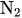?Miki

Concept:

Lewis structure is basically a simplified representation of the structure of any molecule or atom. Lewis structure shows the bonding between the atoms and the lone pairs of electrons as dot.

The steps to draw the Lewis structure of any molecule are as follows:

1. Write the letter symbol for each element present in the compound and draw the skeletal structure. Generally, the least electronegative element is considered as the central element.

2. Count the total number of valence electrons in the molecule. In case of charged molecules subtract the positive charge from the total number of valence electrons and add the negative charge to the total number of valence electrons.

3. Assign two electrons between two atoms and join them via a single bond. Place the remaining valence electrons as lone pairs such that octet of each element is achieved. Use multiple bonds to complete the octet.

Solution:

Step 1: The letter symbol for nitrogen is ‘N’. The skeletal structure ofis as follows: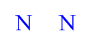Step 2: The electronic configuration of nitrogen is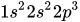. The number of valence electrons for nitrogen atom is 5.

The total number valence electrons inare calculated as follows:Step 3:  Distribute the valence electrons around nitrogen atoms such that the octet for each atom is satisfied.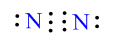The octet of each nitrogen atom is complete. Lewis structure ofis as follows: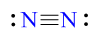Similar problems:

Question:

1.What is the Lewis structure of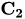?

Solution:

Step 1: The letter symbol for carbon is ‘C’. The skeletal structure ofis as follows: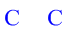Step 2: The electronic configuration of carbon is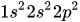. The number of valence electrons for carbon atom is 4.

The total number valence electrons inare calculated as follows:Step 3:  Distribute the valence electrons around carbon atoms such that the octet for each atom is satisfied.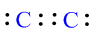The octet of each carbon atom is complete. Lewis structure of  is as follows: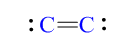Question:

2. What is the Lewis structure of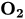?

Solution:

Step 1: The letter symbol for oxygen is ‘O’. The skeletal structure ofis as follows: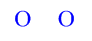Step 2: The electronic configuration of oxygen is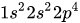. The number of valence electrons for oxygen atom is 6.

The total number valence electrons inare calculated as follows:Step 3:  Distribute the valence electrons around oxygen atoms such that the octet for each atom is satisfied.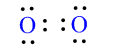The octet of each oxygen atom is complete. Lewis structure of  is as follows: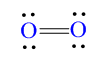Need homework help now?

tutors online

Related Questions
2. (11 marks) a) At 400 °C, ammonium iodide decomposes in the following endothermic reaction: NH41(s) + NH3(g) + HI(g) Kp = 0.215 at 400 °C. Write the equilibrium expression for this reaction. 15.0 g of NH4I was placed in an empty 3.0 L flask and heated to 400 °C. What is the partial pressure of NH3 in the flask once the reaction has reached equilibrium? How would the following changes to the equilibrium mixture affect the number of moles of NH3 in the flask and the value of K, once equilibrium is re-established? (Use increase, decrease or stay the same.) Change Effect on moles of NH3 Ke value Increase temperature Increase volume of flask Remove half of HI gas
Notes
Me

OR

Don't have an account?

Join OneClass

Access over 10 million pages of study
documents for 1.3 million courses.

Join to view

OR

By registering, I agree to the Terms and Privacy Policies
Just a few more details

So we can recommend you notes for your school.